Problem
Suppose f is a differentiable function such that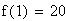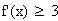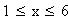What is the smallest possible value for f(6)?
Solution
f is differentiable, so f must be continuous. Therefore, we can apply the Mean Value Theorem on the interval [1, 6]. The theorem assures us there is some number c in the interval such that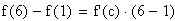so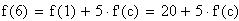Since we are told that f '(x) is at least 3 on this interval, we know that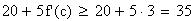Therefore f(6) is greater than or equal to 35, so 35 is the smallest possible value for f(6).